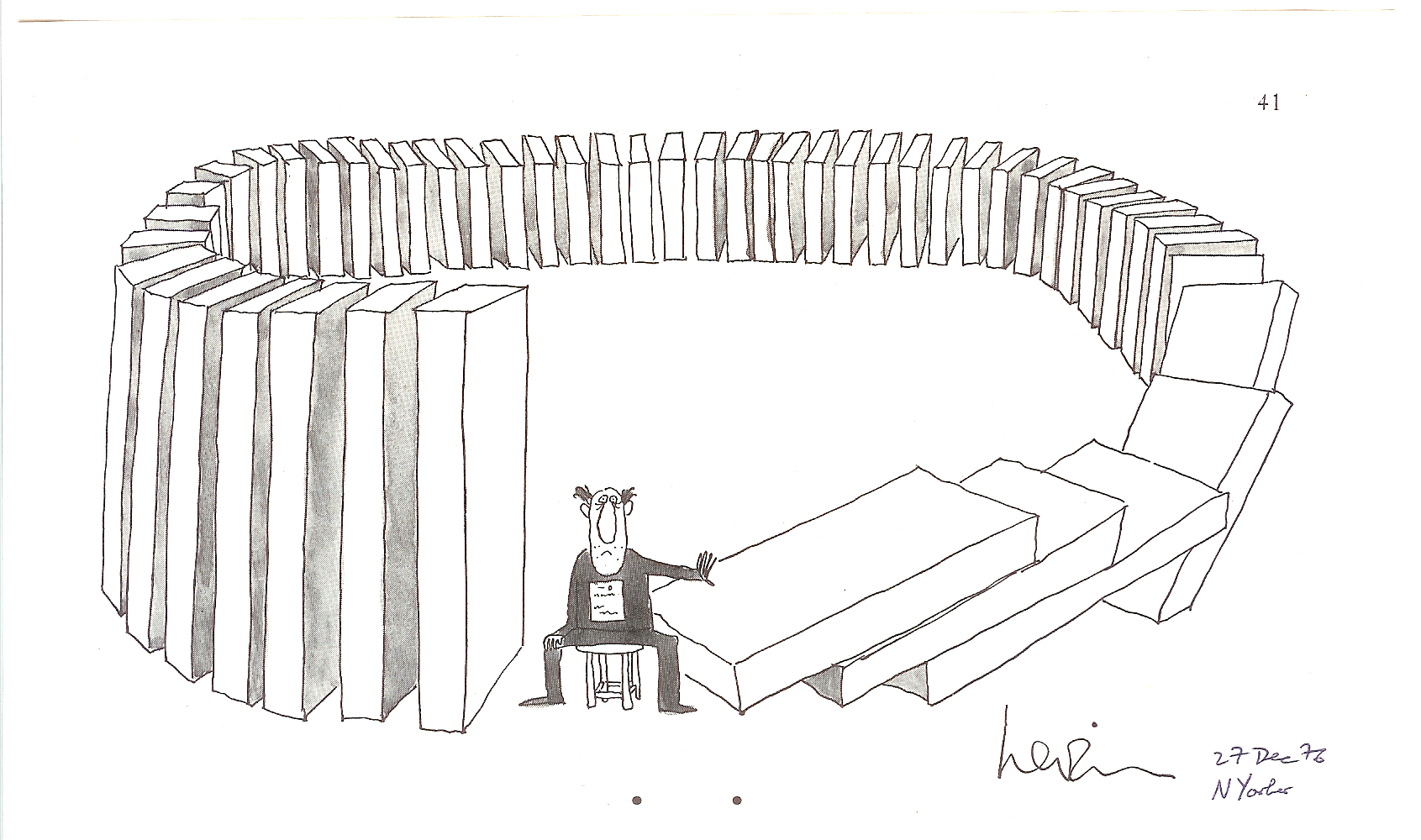# Seductive Recursive Sequences

Algebra Level 2$(\alpha_n)$ and $(\beta_n)$ are two sequences defined recursively as $\alpha_n=2 \alpha_{n-1}+1$ and $\beta_n=8 \beta_{n-1}+1$ with $\alpha_0=\beta_0=0.$ Find the ratio

$\frac{\alpha_{2016}}{\beta_{672}}.$

×